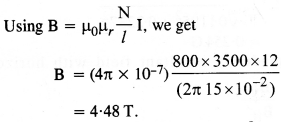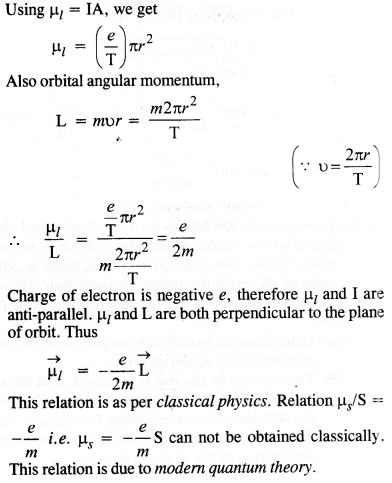# NCERT Solutions Class 12 Physics Chapter-5 (Magnetism and Matter)

NCERT Solutions Class 12 Physics from class 12th Students will get the answers of Chapter-5 (Magnetism and Matter) This chapter will help you to learn the basics and you should expect at least one question in your exam from this chapter.
We have given the answers of all the questions of NCERT Board Physics Textbook in very easy language, which will be very easy for the students to understand and remember so that you can pass with good marks in your examination.Class 12 Physics

Chapter-5 (Magnetism and Matter)

Questions and answers given in practice

Chapter-5 (Magnetism and Matter)

# Question 1.

Answer the following questions regarding earth’s magnetism :
(a) A vector needs three quantities for its specification. Name the three independent quantities conventionally used to specify the earth’s magnetic field.
(b) The angle of dip at a location in southern India is about 18°. Would you expect a greater or smaller dip angle in Britain ?
(c) If you made a map of magnetic field lines at Melbourne in Australia, would the lines seem to go into the ground or come out of the ground ?
(d) In which direction would a compass free to move in the vertical plane point to, if located right on the geomagnetic north or south pole ?
(e) The earth’s field, it is claimed, roughly approximates the field due to a dipole of
magnetic moment 8 x 1022 JT_1 located at its center. Check the order of magnitude of this number in some way.
(f) Geologists claim that besides the main magnetic N­S poles, there are several local poles on the earth’s surface oriented in different directions. How is such a thing possible at all ?

(a) Magnetic elements

• Declination
• Dip and
• Horizontal intensity

(b) Greater in Britain (it is about 70°), because Britain is closer to the magnetic north pole.

(c) Field lines of B due to the earth’s magnetism would seem to come out of the ground.

(d) Compass needle can move only in the horizontal plane. Since the field is entirely vertical no direction is shown by the needle.
(e) Using the formula for magnetic field on the equatorial line of a magnetic dipole i.e.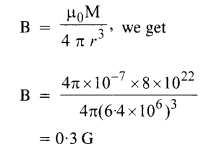This value tells the order of magnitude of magnetic field of earth.
(f) Geologists are correct to think so because it is an approximation to consider the magnetic field of earth to be a single dipole field. The magnetised mineral deposits can be treated as local dipoles on earth.

Question 2.
(a) The earth’s magnetic field varies from point to point in space. Does it also change with time ? If so, on what time scale does it change appreciably ?
(b) The earth’s core is known to contain iron. Yet geologists do not regard this as a source of the earth’s magnetism. Why ?
(c) The charged currents in the outer conducting regions of the earth’s core are thought to be responsible for earth’s magnetism. What might be the ‘battery’ (i.e. the source of energy) to sustain these currents ?
(d) The earth may have even reversed the direction of its field several times during its history of 4 to 5 billion years. How can geologists know about the earth’s field in such distant past ?
(e) The earth’s field departs from its dipole shape substantially at large distances (greater than about 30,000 km). What agencies may be responsible for this distortion ?(f) Interstellar space has an extremely weak magnetic field of the order of 1012 T. Can such a weak field be of any significant consequence ? Explain.

(a) Yes, it changes with time. After a few hundred years, the earth’s magnetic field undergoes an appreciable change.

(b) The temperature inside the earth is so high that it is impossible for the iron to remain as a magnet and act as a source of the magnetic field. The magnetic field due to the earth is considered to be due to the circulating electric currents induced in the iron in the molten state and other conducting materials inside the earth.

(c) A possible explanation can be the phenomenon of radioactivity.

(d) Analysis of the rock magnetism /earth’s magnetic field gets recorded in certain rocks during solidification, (through weekly) provides clues to the geomagnetic history.

(e) At large distances, the earth’s magnetic field gets modified by the fields produced by the motion of ions in the earth’s ionosphere.

(f) At very-very large distances like interstellar distances the small fields can significantly affect the charged particles like that of cosmic rays. For small distances, the deflections are not noticeable for small fields but at very large distances the deflections are significant.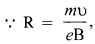clearly small value of B gives a very large value of radius R

Question 3.
A short bar magnet placed with its axis at 30° with a uniform external magnetic field of 0.25 T experiences a torque of magnitude equal to 4.5 X 10-2 J. What is the magnitude of magnetic moment of the magnet ?

Using τ- MB sin θ, we get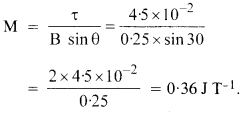Question 4.
A short bar magnet of magnetic moment m = 0.32 JT-1 is placed in a uniform magnetic field of 0.15 T. If the bar is free to rotate in the plane of the field, which orientation would correspond to its (a) stable, and (b) unstable equilibrium ? What is the potential energy of the magnet in each case ?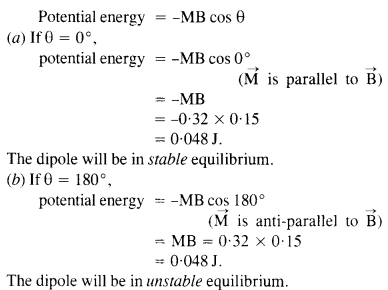Question 5.
A closely wound solenoid of 800 turns and area of cross section 2.5 x 10-4 m2 carries a current of 3.0 A. Explain the sense in which the solenoid acts like a bar magnet. What is its associated magnetic moment ?

When current is passed through the solenoid, the magnetic field is produced along with its axis. The magnetic field lines emanate from one end and enter the other just as in the case of a bar magnet. The two ends of the solenoid act as the two poles of a bar magnet.
Here, the number of turns in the solenoid = 800
I = 3A
A = 2.5 x 10-4m2
The magnetic moment of the solenoid,
M = (IA) x number of turns
= 3 x 2.5 x 10-4 x 800
= 0.6 Am2

Question 6.
If the solenoid in Exercise 5.5 is free to turn about the vertical direction and a uniform horizontal magnetic field of 0.25 T is applied, what is the magnitude of the torque on the solenoid when its axis makes an angle of 30° with the direction of the applied field ?

Using x = MB sin θ, we get
x = 0.6 x 0.25 x sin 30
= 0.6 x 0.25 x= 0.3 x 0.25 = 0.075 Nm
= 7.5 x 10-2 Nm.

Question 7.
A bar magnet of magnetic moment 1.5 JT-1 lies aligned with the direction of a uniform magnetic field of 0.22T.
(a) What is the amount of work required by an external torque to turn the magnet so as to align its magnetic moment, (i) normal to the field direction, (ii) opposite to the field direction ?
(b) What is the torque on the magnet in cases (i) and (ii)?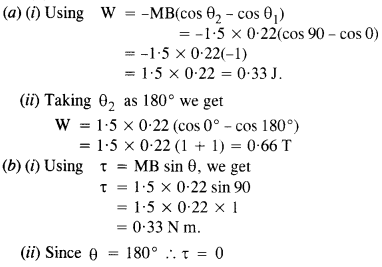Question 8.
A closely wound solenoid of 2000 turns and area of cross-section 1.6 X 10-4 m2, carrying a current of 4.0 A, is suspended through its center allowing it to turn in a horizontal plane.
(a) What is the magnetic moment associated with the solenoid ?
(b) What is the force and torque on the solenoid if a uniform horizontal magnetic field of 7.5 x 10-2 T is set up at an angle of 30° with the axis of the solenoid?

N =2000, A= 1.6 x 10-4m2, I = 4.0 A
(a) m = ANI = 1.6 x 10-4 x 2000 x 4.0
= 1.28 Am2, along the axis

(b) B = 7.5 x 10-2T, θ = 30°
Net force = 0
τ = mB sin θ = 1.28 x 7.5 x 10-2 x sin 30
= 0.64 x 7.5 x 10-2
= 4.800 x 10-2 Nm
By the action of this, the solenoid can come to the direction of the external field.

Question 9.
A circular coil of 16 turns and radius 10 cm carrying a current of 0.75 A rests with its plane normal to an external field of magnitude 5.0 x 10-2 T. The coil is free to turn about an axis in its plane perpendicular to the field direction. When the coil is turned slightly and released, it oscillates about its stable equilibrium with a frequency of 2.0 s_1. What is the moment of inertia of the coil about its axis of rotation ?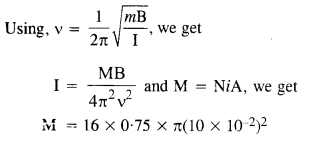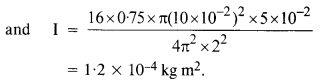Question 10.
A magnetic needle free to rotate in a vertical plane parallel to the magnetic meridian has its north tip pointing down at 22° with the horizontal. The horizontal component of the earth’s magnetic field at the place is known to be 0.35 G. Determine the magnitude of the earth’s magnetic field at the place.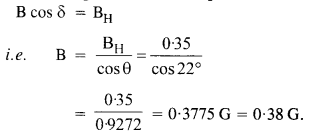Question 11.
At a certain location in Africa, a compass points 12° west of the geographic north. The north tip of the magnetic needle of a dip circle placed in the plane of magnetic meridian points 60° above the horizontal. The horizontal component of the earth’s field is measured to be 0.16 G. Specify the direction and magnitude of the earth’s field at the location.

Using BH = B cos δ, we get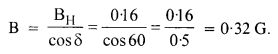Direction of B is 12° west of geographic meridian making upward angle of 60° with horizontal.

Question 12.
A short bar magnet has a magnetic moment of 0.48 JT-1. Give the direction and magnitude of the magnetic field produced by the magnet at a distance of 10 cm from the center of the magnet on (a) the axis, (b) the equatorial lines (normal bisector) of the magnet.

On axial line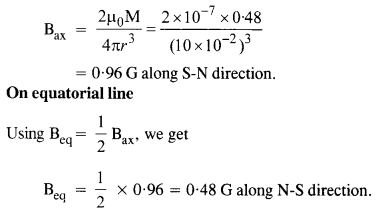Question 13.
A short bar magnet placed in a horizontal plane has its axis aligned along the magnetic north-south direction. Null points are found on the axis of the magnet at 14 cm from the center of the magnet. The earth’s magnetic field at the place is 0.36 G and the angle of dip is zero. What is the total magnetic field on the normal bisector of the magnet at the same distance as the null-points (i.e., 14 cm) from the center of the magnet ? (At null points, Held due to a magnet is equal and opposite to the horizontal component of earth’s magnetic field.)

Magnetic field at the equatorial line of the magnet is given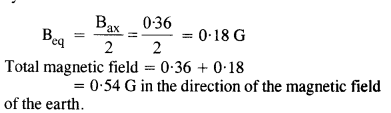Question 14.
If the bar magnet in Exercise 5.13 is turned around by 180°, where will the new null-points be located ?

When magnet is turned around 180°, its south pole lies in the geographical south direction. Hence null point will lie on the equatorial line at a distance x from die center of the magnet.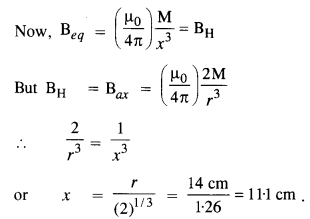Question 15.
A short bar magnet of magnetic moment 5.25 x 10-2 JT-1 is placed with its axis perpendicular to the earth’s field direction. At what distance from the center of the magnet, the resultant field is inclined at 45° with the earth’s field on (a) its normal bisector and (b) its axis. Magnitude of the earth’s field at the place is given to be 0.42 G. Ignore the length of the magnet in comparison to the distances involved.

Normal bisector
(a) Let resultant magnetic field of a magnet at point P makes an angle θ= 45° with the earth’s field. Therefore,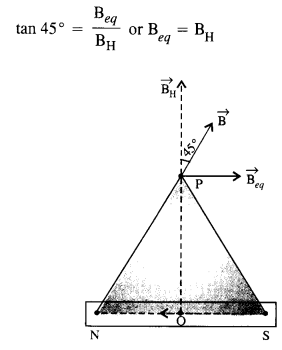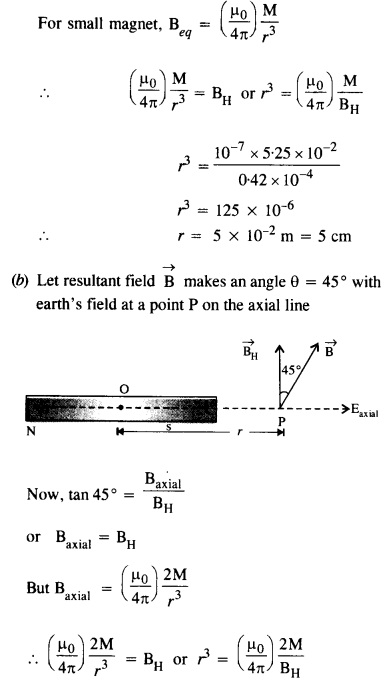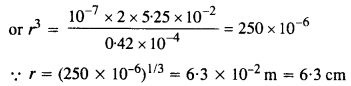Question 16.
(a) Why does a paramagnetic sample display greater magnetisation (for the same magnetising field) when cooled?
(b) Why is diamagnetism, in contrast, almost independent of temperature?
(c) If a toroid uses bismuth for its core, will the field in the core be (slightly) greater or (slightly) less than when the core is empty?
(d) Is the permeability of a ferromagnetic material independent of the magnetic field? If not, is it more for lower or higher fields?
(e) Magnetic field lines are always nearly normal to the surface of a ferromagnet at every point. (This fact is analogous to the static electric field lines being normal to the surface of a conductor at every point). Why?
(f) Would the maximum possible magnetisation of a paramagnetic sample be of the same order of magnitude as the magnetisation of a ferromagnet ?

(a) When cooled, the tendency of the thermal agitation to disrupt the alignment of magnetic dipoles decreases in the case of paramagnetic materials. Hence they display greater magnetisation.

(b) The atoms of a diamagnetic do not have an intrinsic magnetic dipole moment. On placing a diamagnetic sample in a magnetic field, the magnetic moment of the sample is always opposite to the direction of the field. It is not affected by the thermal motion of the dipoles.

(c) Since bismuth is diamagnetic, the field in the core coil be sightly less than that when a core is empty.

(d) Permeability of a ferromagnetic material depends on applied magnetic field. Permeability is more for lower magnetic field.

(e) One of the reasons for this fact is that when a material has µr > > 1, the field lines meet the material nearly normally.

(f) Yes, a paramagnetic sample with saturated magnetisation will have the same .order of magnetisation as the magnetisation of a ferromagnetic substance. However, the saturated magnetisation will require magnetising field too high to achive. Further, there may be a minor difference in the strengths of the atomic dipoles of paramagnetic and ferromagnetic materials.

Question 17.
(a) Explain qualitatively on the basis of domain picture the irreversibility in the magnetisation curve of a ferromagnet.
(b) The hysteresis loop of a soft iron piece has a much smaller area than that of a carbon steel piece. If the material is to go through repeated cycles of magnetization, which piece will dissipate greater heat energy ?
(c) ‘A system displaying a hysteresis loop such as a ferromagnet is a device for storing memory.’ Explain the meaning of this statement.
(d) What kind of ferromagnetic material is used for coating magnetic tapes in a cassette player, or for budding ‘memory stores’ in a modern computer ?
(e) A certain region of space is to be shielded from magnetic fields. Suggest a method.

A piece of carbon steel will dissipate a greater amount of heat energy as its hysteresis loop has a greater area.
The magnetisation produced in a ferromagnet does not have a unique value corresponding to the applied magnetizing field.

In addition, the magnetisation produced depends on the history of the magnetisation i.e. the number of cycles of magnetisation, it has been taken through. In other words, the value of magnetisation of a ferromagnet is a record or memory of its magnetisation. If information bits can be made corresponding to the cycles of magnetization, the system displaying the hysteresis loop of the ferromagnet can act as a device for storing the information.

• ceramics are used for coating magnetic tapes in a cassette player or for building memory stores in a modem computer. Ceramics are specially treated barium iron oxides and are also called ferrates.
• The shielding of the region can be done by surrounding it with soft iron rings. The magnetic field lines will be drawn into the rings and the enclosed region will become free of the magnetic field.

Question 18.
A long straight horizontal cable carries a current of 2.5 A in the direction 10° south of west to 10° north of east. The magnetic meridian of the place happens to be 10° west of the geographic meridian. The earth’s magnetic field at the location is 0.33 G, and the angle of dip is zero. Locate the line of neutral points (Ignore the thickness of the cable). (At neutral points, magnetic field due to a current-carrying cable is equal and opposite to the horizontal component of earth’s magnetic field.)

Let neutral point lies at a distance x from the cable. Now, at neutral point, magnetic field due to cable is equal in magnitude and opposite in direction of the earth’s magnetic field.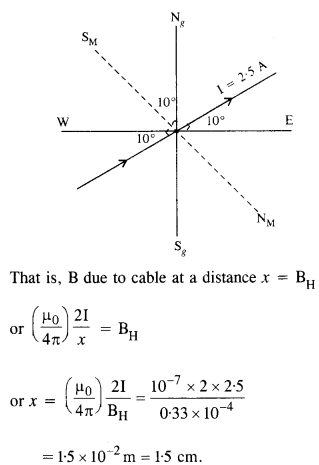Question 19.
A telephone cable at a place has four long straight horizontal wires carrying a current of 1.0 A in the same direction east to west. The earth’s magnetic field at the place is 0.39 G, and the angle of dip is 35°. The magnetic declination is nearly zero. What are the resultant magnetic fields at points 4.0 cm below the cable?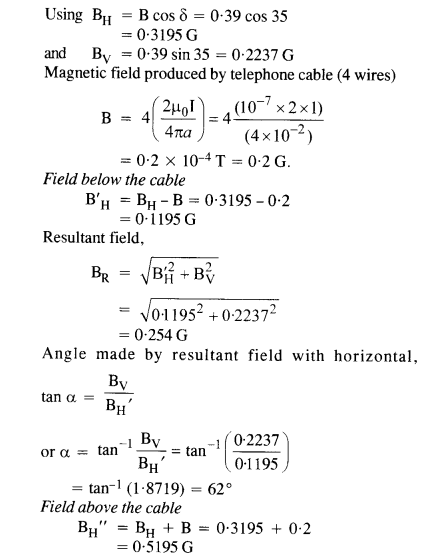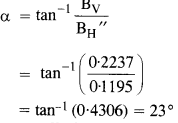Question 20.
A compass needle free to turn in a horizontal plane is placed at the center of circular coil of 30 turns and radius 12 cm. The coil is in a vertical plane making an angle of 45° with the magnetic meridian. When the current in the coil is 0.35 A, the needle points west to east.
(a) Determine the horizontal component of the earth’s magnetic field at the location.
(b) The current in the coil is reversed, and the coil is rotated about its vertical axis by an angle of 90° in the anticlockwise sense looking from above. Predict the direction of the needle. Take the magnetic declination at the place to be zero.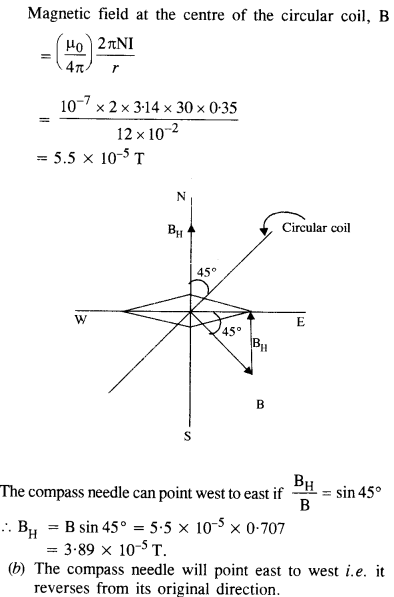Question 21.
A magnetic dipole is under the influence of two magnetic fields. The angle between the field directions is 60°, and one of the fields has a magnitude of 1.2 x 10-2 T. If the dipole comes to stable equilibrium at an angle of 15° with this field, what is the magnitude of the other field ?

Here B, = 1.2 X 10-2 T,θ1= 15°, θ2 = 45°.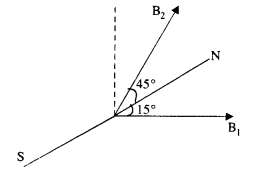The dipole will be in equilibrium, if torque acting on dipole due to B1 is equal and opposite to the torque acting on dipole due to B2.
That is, MBsin = MB2 sin θ2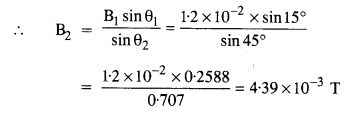Question 22.
A monoenergetic (18 keV) electron beam intially in the horizontal direction is subjected to a horizontal magnetic field of 0.40 G normal to the initial direction. Estimate the up or down deflection of the beam over a distance of 30 cm (me = 9.11 x 10-19 Q.
[Note. Data in this exercise are so chosen that the answer will give you an idea of the effect of earth’s magnetic field on the motion of the electron beam from the electron gun to the screen in a TV set.]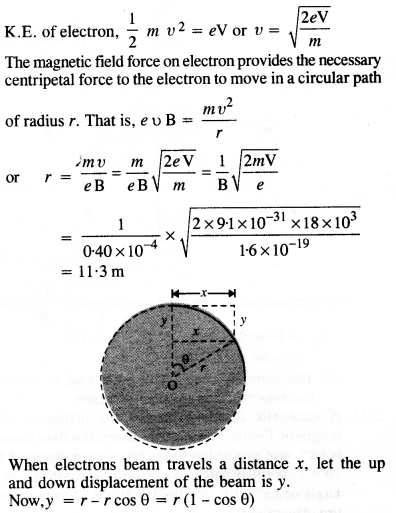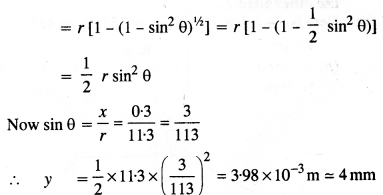Question 23.
A sample of paramagnetic salt contains 2.0 x 1024 atomic dipoles each of dipole moment 1.5 x 10-23 JT-1. The sample is placed under a homogeneous magnetic field of 0.64 T and cooled to a temperature of 4.2 K. The degree of magnetic saturation achieved is equal to 15%. What is the total dipole moment of the sample for a magnetic field of 0.98 T and a temperature of 2.8 K ? (Assume Curie’s law).

Magnetic dipole moment of sample,
M = 15% of M (1.5 x 10-23) (2 x 1024) = 30 JT-1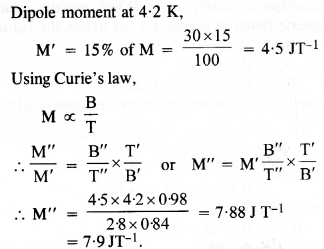Question 24.
A Rowland ring of mean radius 15 cm has 3500 turns of wire wound on a ferromagnetic core of relative permeability 800. What is the magnetic field B in the core for a magnetizing current of 1.2 A?